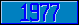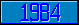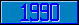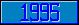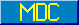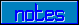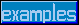## \$%REPLACE^STRING

### M[UMPS] by Example

Approved for inclusion in a future M[UMPS] language standard as part of the library for String Handling.

This function returns a translated version of the value of its parameter.

Suppose that it is necessary to print German umlauted characters on a printer that is not able to produce such characters, in such a case the Umlaut on the character is usually replaced by the vowel "e" following the character. Hence:

Kill SPEC
Set SPEC(1,1)="ä",SPEC(1,2)="ae"
Set SPEC(2,1)="ö",SPEC(2,2)="oe"
Set SPEC(3,1)="ü",SPEC(3,2)="ue"
Set SPEC(4,1)="Ä",SPEC(4,2)="AE"
Set SPEC(5,1)="Ö",SPEC(5,2)="OE"
Set SPEC(6,1)="Ü",SPEC(6,2)="UE"

Write \$%REPLACE^STRING(X,.SPEC)

would write the text in X, with all umlauted characters translated into two characters.

The same function could be used for simplifying output-strings containing device-dependent functions:

Kill S
Set S(1,1)="<UP-ARROW>",S(1,2)=\$Char(27)_"[A"
Set S(2,1)="<DOWN-ARROW>",S(2,2)=\$Char(27)_"[B"
Set S(3,1)="<RIGHT-ARROW>",S(3,2)=\$Char(27)_"[C"
Set S(4,1)="<LEFT-ARROW>",S(4,2)=\$Char(27)_"[D"
Set S(5,1)="<ESCAPE>",S(5,2)=\$Char(27)
Set S(6,1)="<LINE-FEED>",S(6,2)=\$Char(10)
Set X=\$%REPLACE^STRING(INPUT,.S)

would produce a value in X with some human readable device control commands translated into the code-sequences that would be sent to an external device.

The MDC has approved code to approximate the return value of this function. Implementors are encouraged to provide more accurate and efficient code).